hackernoon.com Go back Open original

# 7 Types of Operators in PythonOperators are used to doing operations on any given data stored inside variables. In Python, we learn 7 types of operators - namely :

1. Arithmetic operators
2. Bitwise operators
3. Comparison operators
4. Assignment operators
5. Logical operators
6. Identity operators
7. Membership operators
8. 1. Arithmetic Operators

Arithmetic operators make mathematical operations possible on operands in a program.

I know! I know! This is a basic concept! But let’s make it fun!

## Starting with addition and subtraction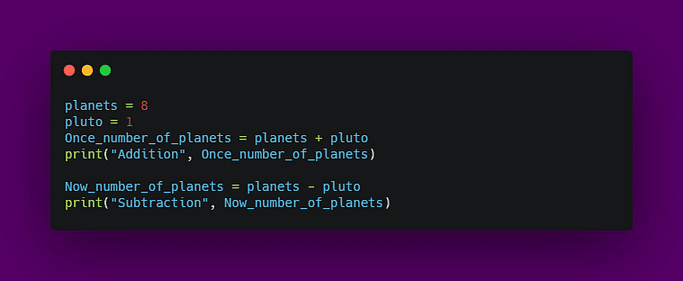Output:

## Now, multiplication and division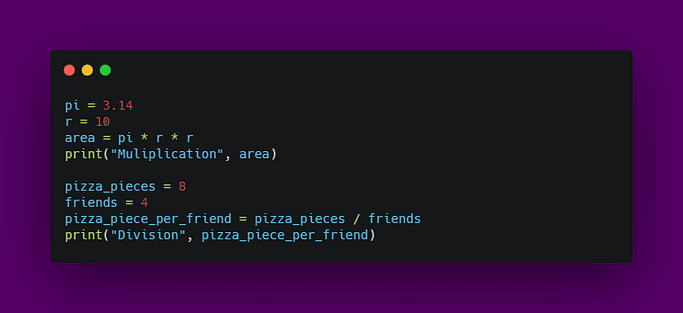Output:

``````Muliplication 314.0
Division 2.0``````

## Special Arithmetic Operators

Floor division — // : rounds off the result to the nearest whole number.

Modulus — % : produces the remainder of the numbers.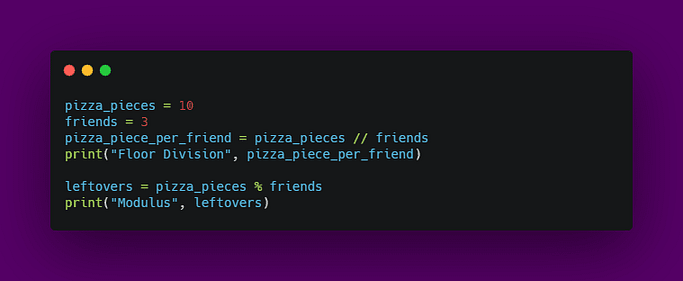Output:

``````Floor Division 3
Modulus 1``````

Exponentiation — **: produces the power of given numbers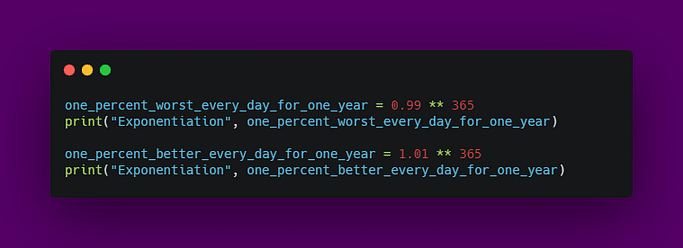Output:

``````Exponentiation 0.025517964452291125
Exponentiation 37.78343433288728``````

## 2. Bitwise Operators in Python

When it comes to binary numbers, bitwise operators are the choice.

Bitwise operators are used to perform operations on binary numbers.

AND, OR, XOR operators

• AND & operator sets each bit to 1 if both bits are 1.
• OR | operator sets each bit to 1 if one of two bits is 1.
• XOR ^ operator sets each bit to 1 if only one of two bits is 1.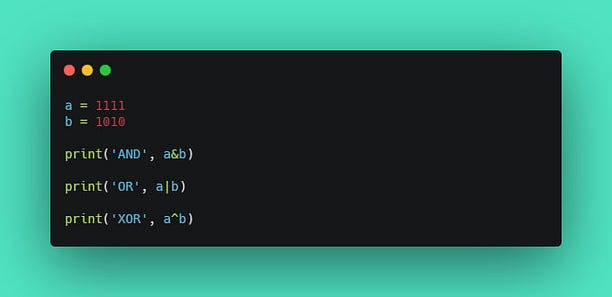Output:

Ha Ha, surprised about the outputs?!

The outputs are a result of the binary numbers a and b which gets converted into an integer, each time bitwise operation is performed.

## NOT operator

NOT ~ operator inverts all the bits. In Python, the number gets converted into an inverted signed number.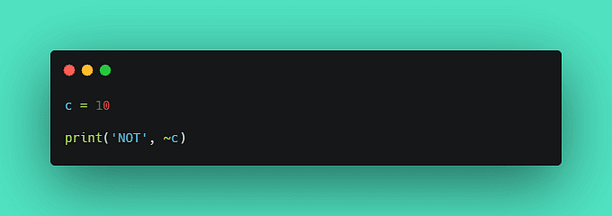Output:

## Shift Operators

• left shift << operator shifts left by pushing zeros in from the right and let the leftmost bits fall off.
• right shift >> operator shifts right by pushing copies of the leftmost bit in from the left, and let the rightmost bits fall off.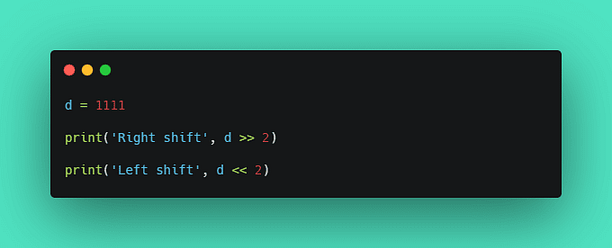Output:

``````Right shift 277
Left shift 4444``````

## 3. Comparison Operators in Python

So, basically, comparison operators are used to compare two values that are numbers.

If we level up to be geeky, comparison operators can also be used to compare other data types.

Now, let’s start with equality checks and I hope you like spider-man movies.

== Equal comparison operator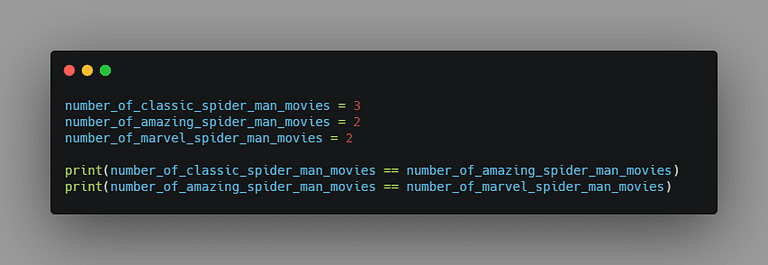Output:

!= Not Equal comparison operator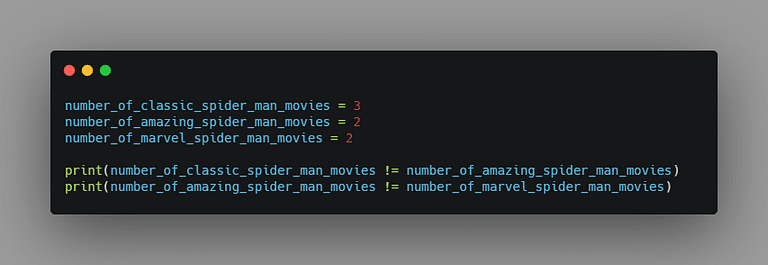Output:

Alright, I’m sure that you are aware of how to use other operators to compare two number values, right? OK, now’s the time to level up to be geeky.

For the rest of the operators let us compare the letters from the Alphabet.
Wait, what?! You heard me right!

Let me explain it at the end of this post.

> Greater than comparison operator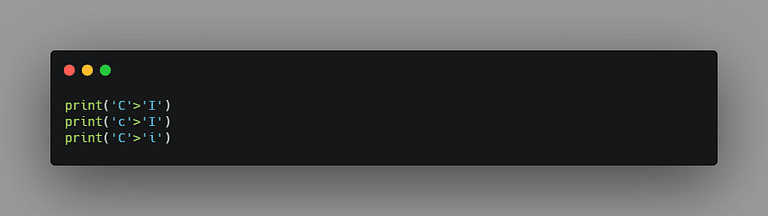Output:

< Less than comparison operator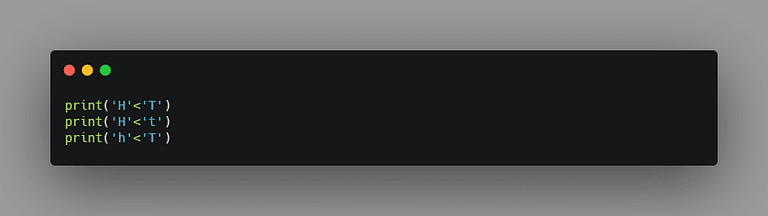Output:

>= Greater than or equal to comparison operator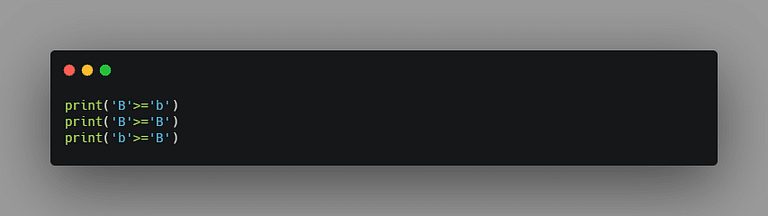Output:

<= Less than or equal to comparison operator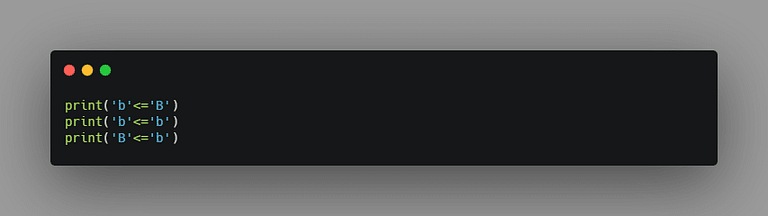Output:

Here’s the answer for the above craziness.

When we compare two letters (or characters), it gets converted into ASCII code. You can check the link where the table contains ‘DEC’ (Decimal values) for the characters from Alphabet.

Now that the characters are converted into ASCII code, which is nothing but numbers, we are back to square one. That is, we can compare the values as numbers and return true or false.

## 4. Assignment Operators in Python

Assignment operators are used to assign values to variables.

That is to store values in variables we use = assignment operator.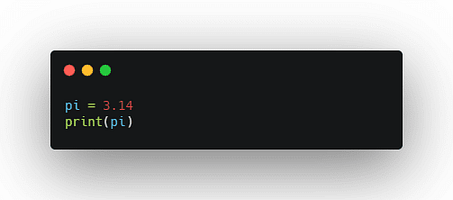Output:

OK, now comes the real fun. Have ever been tired to use x = x + 5, where we type the variable x twice? There's actually a shortcut for this called augmented assignment operators.

Augmented assignment operators can be used as a replacement as follows:

``x += 3 ---> x = x + 3 x -= 3 ---> x = x - 3 x *= 3 ---> x = x * 3 x /= 3 ---> x = x / 3 x %= 3 ---> x = x % 3 x //= 3 ---> x = x // 3 x **= 3 ---> x = x ** 3 x &= 3 ---> x = x & 3 x |= 3 ---> x = x | 3 x ^= 3 ---> x = x ^ 3 x >>= 3 ---> x = x >> 3 x <<= 3 ---> x = x << 3``

Here is the Code and Output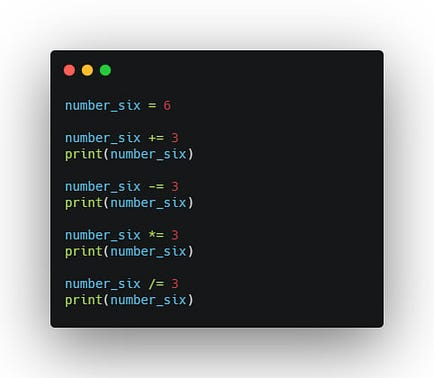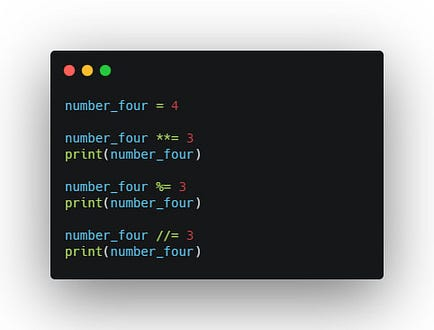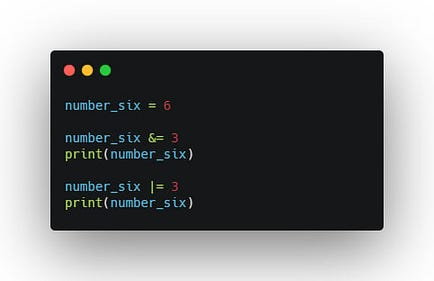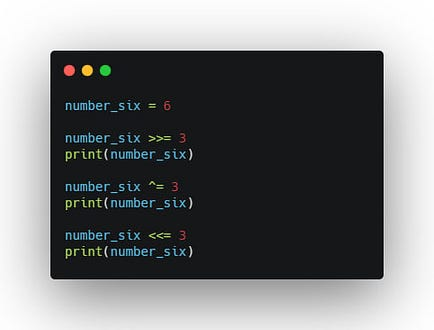Quick Note: The code snippets reuses the same variable to assign with different arithmetic operations / bitwise operations / shift operations.

So, while coding make sure you practice to use print statements after each operation.

## 5. Logical Operators in Python

Logical operators are used to combine more than two conditional statements.

These operators are very useful for writing logical conditions in control flow statements of a programming language.

Let’s code them one by one.

And Operator

and operator returns the boolean value True only if both statements are true.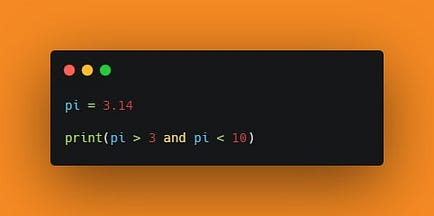Output:

Or Operator

or operator returns the boolean value True if any statement is true.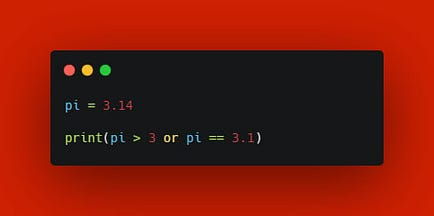NOT operator

not operator acts as a unary operator which returns the boolean value True the statement is true and vice versa.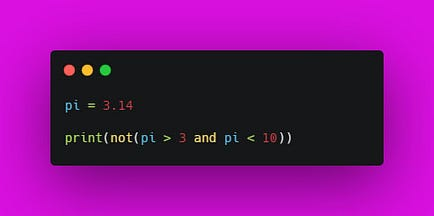Output:

## 6. Identity Operators in Python

Identity operators are used to checking whether the objects are the same or not.

Fact: In python, all data types are implemented as an object.

Operator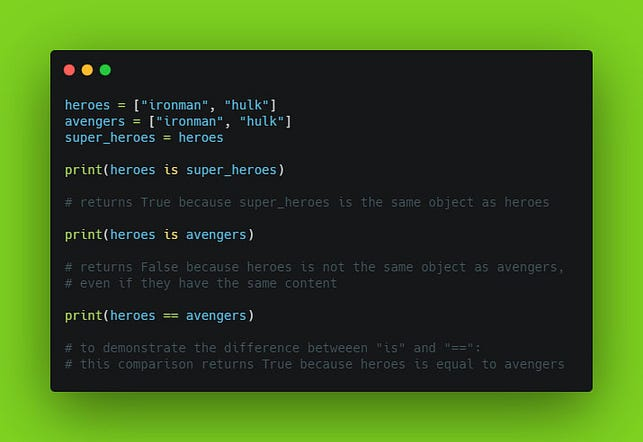Output:

NOT Operator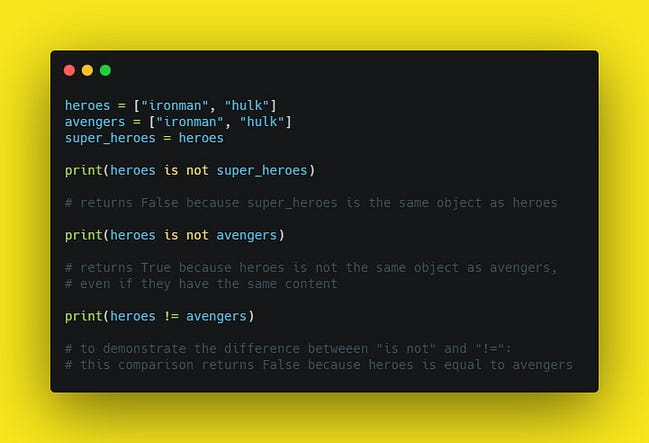Output:

## 7. Membership Operators in Python

Membership operators are used to testing whether a sequence with the specified value is present in the given object.

Fact: In Python, all data types are implemented as an object.

Let’s go code through each of them.

in operator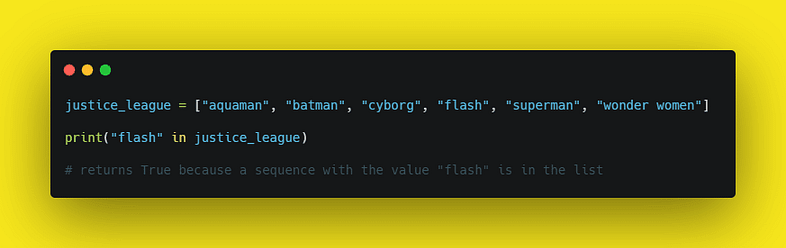Output:

not in operator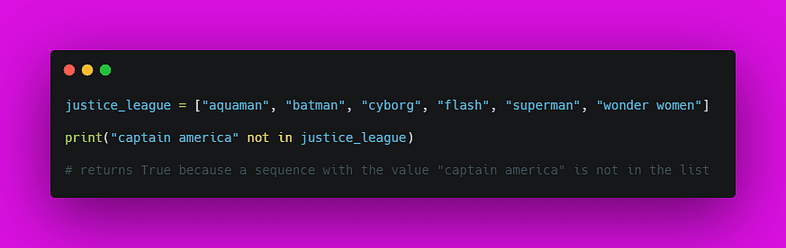Output:

Code along and have fun ;)

Join Hacker Noon

Create your free account to unlock your custom reading experience.